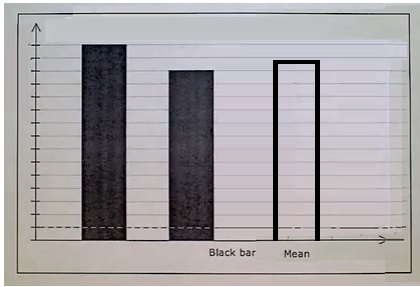# Understanding the Mean Graphically: Two bars

In this lesson we understand the mean of a dataset using graphical method.

Suppose we are given a bar graph showing two bars of data. Here we are required to find the mean of given data graphically.

## Rules to find the mean graphically

• In the bar graph we find the heights of the two bars.

• The average or mean of these heights is found.

• We then draw a third bar with the average height found in second step.

• The height of this third bar gives the mean or average of given data set graphically.The two bars in a bar graph have heights 16 and 22. What height a new bar should have so that it has the mean height of the two bars?

### Solution

Step 1:

Heights of given bars 16, 22

Step 2:

Mean height = $\frac{(16 + 22)}{2} = \frac{38}{2}$ = 19

So height of new bar = 19

The two bars in a bar graph have heights 15 and 27. What height a new bar should have so that it has the mean height of the two bars?

### Solution

Step 1:

Heights of given bars 15, 27

Step 2:

Mean height = $\frac{(15 + 27)}{2} = \frac{42}{2}$ = 21

So height of new bar = 21